# CBSE Class 10 Science MCQ Chapter 12 Electricity

## Electricity Class 10 MCQ

CBSE Class 10 Science MCQ Chapter 12 Electricity is available for free downloading in pdf format for students’ exam preparation. The MCQs have been recently introduced in the exam pattern. So, to help students tackle Electricity MCQ, we have provided a total of 30 questions of Chapter 12 Electricity. By practising them, students can easily answer the MCQs asked from this chapter in the exam. These questions are designed as per the CBSE Class 10 Science Syllabus and exam pattern.

Students must cover the following concepts from the NCERT Class 10 Science Textbook before solving these multiple choice questions to answer them correctly.

• Electric current & circuit
• Electric Potential and Potential Difference
• Circuit Diagram
• Ohm’s Law
• Factors on which resistance depends
• Resistance of a system of resistors
• Heating effect of electric current
• Electric Power

Students can also access the CBSE Class 10 Science MCQs for other chapters to prepare for their board exams.

## CBSE Class 10 Science MCQ Chapter 12 Electricity with Answers

Q1) The image shows a circuit diagram.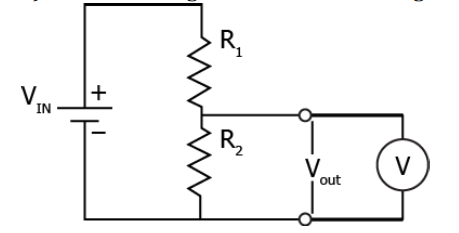What is being measured using the voltmeter?

(a) current in the circuit

(b) voltage in the circuit

(c) voltage across the resistor

(d) resistance offered by the resistor

Q2) The least resistance obtained by using 2 Ω, 4 Ω, 1 Ω and 100 Ω is

(a) < 100 Ω

(b) < 4 Ω

(c) < 1 Ω

(d) > 2 Ω

Q3) Work of 14 J is done to move 2 C charge between two points on a conducting wire. What is the potential difference between the two points?

(a) 28 V

(b) 14 V

(c) 7 V

(d) 3.5 V

Q4) A fuse wire repeatedly gets burnt when used with a good heater. It is advised to use a fuse wire of

(a) more length

(c) less length

Q5) A circuit has a charge of 2C moving through it in 3 s. Which electrical component in the circuit, if present, will show the current?

(a) Voltmeter will show a current of 6 A.

(b) Ammeter will show a current of 0.7 A.

(c) Rheostat will show a current of 0.7 A.

(d) Resistor will show a current of 0.35 A.

Q6) Electrical resistivity of a given metallic wire depends upon

(a) its length

(b) its thickness

(c) its shape

(d) nature of the material

Q7) Two devices are connected between two points, say A and B in parallel. The physical quantity that will remain the same between the two points is

(a) current

(b) voltage

(c) resistance

(d) None of these

Q8) Unit of electric power may also be expressed as

(a) volt ampere

(b) kilowatt hour

(c) watt second

(d) joule second

Q9) What is the relationship between resistance and current?

(a) They are directly related to each other.

(b) They are inversely related to each other.

(c) The resistance has a greater magnitude than current.

(d) The current has a greater magnitude than resistance.

Q10) The resistance whose V – I graph is given below is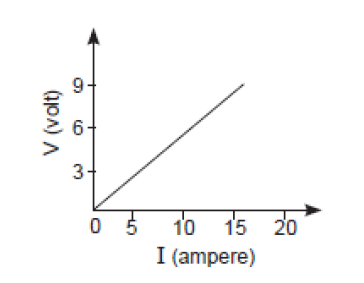(a) 5/3 Ω

(b) 3/5 Ω

(c) 5/2 Ω

(d) 2/5 Ω

Q11) A current of 1 A is drawn by a filament of an electric bulb. Number of electrons passing through a cross section of the filament in 16 seconds would be roughly

(a) 1020

(b) 1016

(c) 1018

(d) 1023

Q12) How much more heat is produced, if the current is doubled?

(a) twice the original amount

(b) thrice the original amount

(c) four times the original amount

(d) five times the original amount

Q13) Which of the following represents voltage?

(a) Work done / Current×Time

(b) Work done × Charge

(c) Work done×Time / Current

(d) Work done × Charge × Time

Q14) A cooler of 1500 W, 200 volt and a fan of 500 W, 200 volt are to be used from a household supply. The rating of fuse to be used is

(a) 2.5 A

(b) 5.0 A

(c) 7.5 A

(d) 10 A

Q15) Which combination of a 2 Ω resistor and 4 Ω resistor offers the least resistance to current in the circuit?

(a) Series combination, which results in a net resistance of 2 Ω.

(b) Parallel combination, which results in a net resistance of 2 Ω.

(c) Series combination, which results in a net resistance of 1.5 Ω.

(d) Parallel combination, which results in a net resistance of 0.5 Ω.

Q16) In an electrical circuit two resistors of 2 Ω and 4 Ω, respectively are connected in series to a 6 V battery. The heat dissipated by the 4 Ω resistor in 5 s will be

(a) 5 J

(b) 10 J

(c) 20 J

(d) 30 J

Q17) In order to reduce electricity consumption at home, what kind of appliance should one purchase?

(a) one which draws low power

(b) one which produces less heat

(c) one which operates at a higher voltage

(d) one which draws a high amount of current

Q18) If n resistors each of resistance R are connected in parallel combination then their equivalent resistance is

(a) R/n2

(b) n2/R

(c) n/R

(d) R/n

Q19) Which one among a bar of an alloy of mass 2 kg and a 3 kg iron bar of same dimension has greater resistivity?

(a) Iron bar because it has higher mass.

(b) Alloy bar because it has lower mass.

(c) Iron bar because it has the same types of atoms.

(d) Alloy bar because it has different types of atoms.

Q20) Two resistors connected in series give an equivalent resistance of 10 Ω. When connected in parallel, give 2.4 Ω. Then the individual resistance is

(a) each of 5 Ω

(b) 6 Ω and 4 Ω

(c) 7 Ω and 4 Ω

(d) 8 Ω and 2 Ω

Q21) A battery of 10 volt carries 20,000 C of charge through a resistance of 20 Ω. The work done in 10 seconds is

(a) 2 × 103 joule

(b) 2 × 105 joule

(c) 2 × 104 joule

(d) 2 × 102 joule

Q22) Two bulbs are rated 40W, 220W and 60W, 220W. The ratio of their resistances will be:

(a) 4:3

(b) 3:4

(c) 2:3

(d) 3:2

Q23) The image shows a combination of 4 resistors.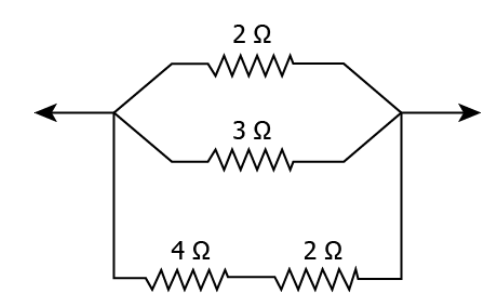What is the net resistance between the two points in the circuit?

(a) 0.5 Ω

(b) 1.0 Ω

(c) 1.5 Ω

(d) 2.0 Ω

Q24) If R1 and R2 be the resistance of the filament of 40 W and 60 W, respectively operating 220 V, then

(a) R1 < R2

(b) R2 < R1

(c) R1 = R2

(d) R1 ≥ R2

Q25) An electric toaster has a power rating of 200 W. It operates for 1 hour in the morning and 1 hour in the evening. How much does it cost to operate the toaster for 10 days at Rs 5 per kW h?

(a) Rs 20

(b) Rs 400

(c) Rs 5000

(d) Rs 10000

Q26) A coil in the heater consumes power P on passing current. If it is cut into halves and joined in parallel, it will consume power

(a) P

(b) P/2

(c) 2 P

(d) 4 P

Q27) Calculate the current flow through the 10 Ω resistor in the following circuit.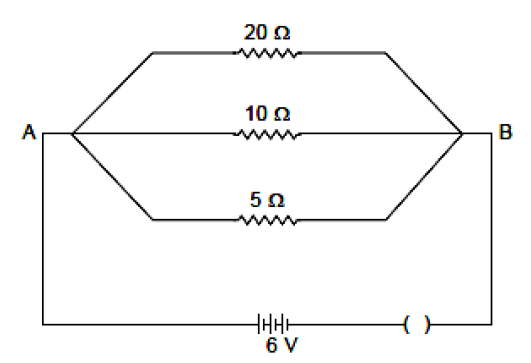(a) 1.2 A

(b) 0.6 A

(c) 0.2 A

(d) 2.0 A

Q28) The effective resistance between A and B is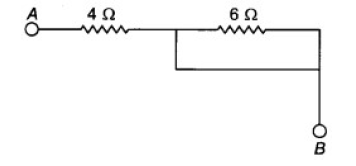(a) 4Ω

(b) 6Ω

(c) May be 10 Ω

(d) Must be 10 Ω

Q29) In this question, the Assertion and Reason have been put forward. Read the statements carefully and choose the correct alternative from the following:

Assertion: In an open circuit, the current passes from one terminal of the electric cell to another.

Reason: Generally, the metal disc of a cell acts as a positive terminal.

(a) Both the Assertion and the Reason are correct and the Reason is the correct explanation of the Assertion.

(b) The Assertion and the Reason are correct but the Reason is not the correct explanation of the Assertion.

(c) Assertion is true but the Reason is false.

(d) The statement of the Assertion is false but the Reason is true.

## Frequently Asked Questions on Electricity

Ohm-meter.

Voltmeter.

### A wire of resistance R is cut into ten equal parts which are then joined in parallel. What will be the new resistance?

0.01R

We hope students have found this information on “CBSE Class 10 Science MCQ Chapter 12 Electricity” useful for their exam preparation. Keep learning and stay tuned for further updates on the CBSE Exam. Download BYJU’S App and subscribe to the YouTube channel to access interactive study videos.